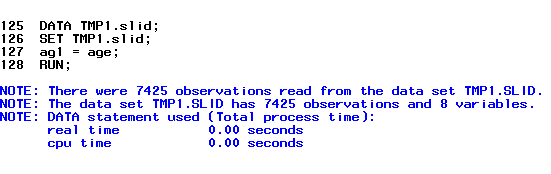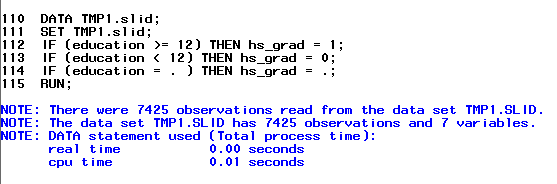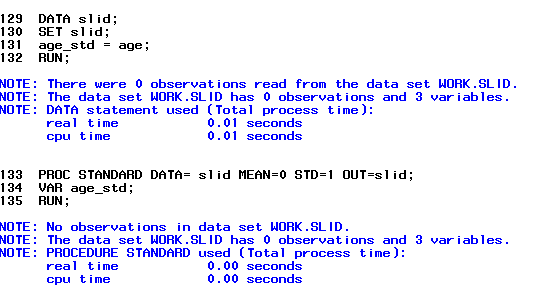It looks like you're using Internet Explorer 11 or older. This website works best with modern browsers such as the latest versions of Chrome, Firefox, Safari, and Edge. If you continue with this browser, you may see unexpected results.

# SAS

## Methods for Creating and Transforming Variables

Generating variables in Stata is quite simple, especially if you want to generate a new variable from an already existing variable. Researchers often generate new variables that are copies of a current one if they want to change or recode the data, while also keeping the original data so it is not lost. There is no formula for generating a new variable as it is likened to “copy” and “paste”.

Below is the code for generating the variable age1 from an already existing variable age

Code

DATA slid;
SET slid;
age1 = age;
RUN

We are using the DATA slid, where our new variable age1 is equal to the already existing variable age.  We then end with the RUN command.

Below is the output for generating a new variable that is a copy of already existing data.

OutputThe output is simply the code above. Sas only gives us this output to tell us the code ran correctly and there are no issues. Another way to check is to go dataset tab in Sas and look for our new variable age1 as an added column.

We can also generate new variables that are transformed from other variables in the dataset. This is helpful if we want to collapse a variable from a higher level of measurement to a lower level of measurement, such as continuous to categorical.

Below is the code for generating the new variable highschool from the variable education that is recoded from a continuous level to a categorical level.

Code

DATA slid;
SET slid;
IF (education >= 12) THEN highschool = 1
IF (education < 12) THEN highschool = 0
IF (education = . ) THEN highschool = .
RUN;

We are using the DATA slid to create the variable highschool that is equal to 1 IF the values of education are equal to or greater than 12. IF education is less than 12 THEN highschool is equal to 0. The last line of code is to make sure all missing values in education remain missing in highschool.

Output

The output for this is similar to the previous example. A copy of the code is shown, the number of observations read and generated from the IF and THEN statements are shown. Sas only gives us this output to tell us the code ran correctly and there are no issues. Another way to check is to go dataset tab in Sas and look for our new variable highschool as an added column.Standardizing a variable from raw values to standard values is often done for variables that do not have a normal distribution. In this case, we are standardizing the variable age in years to Z scores.

Below is the code that will create a new variable called age_std which will have the standardized Z scores of age

Code

DATA slid;
SET slid;
age_std = age;
RUN

PROC STANDARD DATA= slid MEAN=0 STD=1 OUT=slid;
VAR age_std
RUN

OutputSas only gives us this output to tell us the code ran correctly and there are no issues. Another way to check is to go dataset tab in Sas and look for our new variable age_std as an added column.